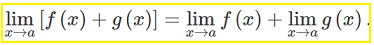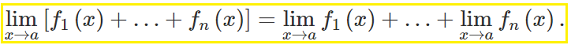# Sum Rule

In Calculus, the “sum rule” is actually a set of three different rules, depending on what you’re using it for. Click on a section to skip to the specific rule you need:

## Sum Rule (also called Sum of functions rule) for LimitsThe limit of a sum equals the sum of the limits. In other words, figure out the limit for each piece, then add them together.

Example: Find the limit as x→2 for x2 + 5

1. The limit of x2 as x→2 (using direct substitution) is x2 = 22 = 4
2. The limit of the constant 5 (rule 1 above) is 5
3. Add (1) and (2) together: 4 + 5 = 9

Note that there’s another sum rule in calculus, and it’s to do with integration. Don’t get confused between the two (if you’re dealing with limits, stick to this page, but for integrals, see: Sum Rule: Definition and Examples).

## Extended Sum Rule:All this is saying is that you can keep on adding limits to the sum rule.

Example: Find the limit as x→2 for x2 + 5 + 10x

1. The limit of x2 as x→2 (using direct substitution) is x2 = 22 = 4
2. The limit of the constant 5 (rule 1 above) is 5
3. Limit of 10x (using direct substitution again) = 10(2) = 20
4. Add (1), (2) and (3) together: 4 + 5 + 20 = 29

## Derivatives

The sum rule for derivatives states that if you have two functions f + g, then the derivative is simply the sum of the two derivatives: f’ + g’.

Example question: What is the derivative of y = x + x2?

Step 1:Find the individual derivatives (ignoring the + symbol). For this example, you have two derivatives: x and x2:

• Derivative of y = x is y’ = 1
• Derivative of y = x2 is y’ = 2x

Step 2:Add the derivatives from Step 1. You aren’t actually summing them (as in 1 + 2 = 3), but rather you just place an “+” symbol between them (as in 1 + 2):
Adding those together, we get y = 1 + 2x

## Sum Rule for Integration

The sum rule states that if you have two functions, the sum (additions) of their integrals always equals to the integral of their sum. In other words, if you have a string of additive functions, you can integrate them term by term.

This rule applies to both definite integrals and indefinite integrals

## Sum Rule for Indefinite Integrals

For indefinite integrals, the sum rule is:

Where:

• f(x) is the function being integrated (the integrand),
• dx is the variable with respect to which we are integrating.

## Example

Integrate the following expression using the sum rule:Step 1: Rewrite the equation into two integrals:

∫(4x2 + 1)/dx becomes:

• ∫4x2 dx +
• ∫ 1 dx

Step 2: Use the usual rules of integration to integrate each part.

∫4x2 dx

• Move the constant factor outside of the integration symbol (See: multiplication rule for indefinite integrals):• Apply the power functions rule for integration. Basically, you add 1 to you exponent, then divide by the new exponent:∫ 1 dx

• Here, the integral of a constant is that constant multiplied by x:
∫ x dx = 1 * x = x.

Step 3: Sum up all of the parts, and add a constant:## Sum Rule for Definite Integrals

For definite integrals, the sum rule is different (but not by much). The main difference is that after you follow the steps above for finding the indefinite integral, you have to calculate the values at the limits of integration.

As an example, let’s add the boundary of 0 to 4 to the above problem:Step 1: Find the indefinite integral. For this particular example, we already calculated the indefinite integral above, which was:## Calculating the Bounds

In the next part, which differs from the steps above for the indefinite integral, you’re looking for a specific area. For this example, it’s the area between 0 and 4 on the graph (i.e. the bounds of integration specified in the problem):Step 1: Calculate the integral at the upper bound (which is 4):Step 2: Calculate the integral at the lower bound (which is 0):Step 3: Calculate the final answer by subtracting the parts (upper bound – lower bound):That’s it!

CITE THIS AS:
Stephanie Glen. "Sum Rule" From StatisticsHowTo.com: Elementary Statistics for the rest of us! https://www.statisticshowto.com/derivatives/sum-rule/